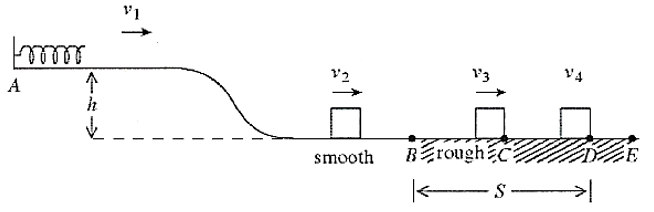Problem: A 1.86-kg block is held in place against the spring by an 81-N horizontal external force (see the figure). The external force is removed, and the block is projected with a velocity v1 = 1.2 m/s upon separation from the spring. The block descends a ramp and has a velocity v2 = 1.9 m/s at the bottom. The track is frictionless between points A and B. The block enters a rough section at B, extending to E. The coefficient of kinetic friction over this section is 0.28. The velocity of the block is v3 = 1.4 m/s at C. The block moves on to D, where it stops. The height h of the ramp is closest to _____. A. 15 cm B. 17 cm C. 11 cm D. 18 cm E. 7.3 cm

FREE Expert Solution
83% (286 ratings)
Problem Details

A 1.86-kg block is held in place against the spring by an 81-N horizontal external force (see the figure). The external force is removed, and the block is projected with a velocity v1 = 1.2 m/s upon separation from the spring. The block descends a ramp and has a velocity v2 = 1.9 m/s at the bottom. The track is frictionless between points A and B. The block enters a rough section at B, extending to E. The coefficient of kinetic friction over this section is 0.28. The velocity of the block is v3 = 1.4 m/s at C. The block moves on to D, where it stops. The height h of the ramp is closest to _____.

A. 15 cm

B. 17 cm

C. 11 cm

D. 18 cm

E. 7.3 cm